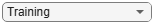# predict

Compute conditional PD

## Syntax

``conditionalPD = predict(pdModel,data)``

## Description

example

````conditionalPD = predict(pdModel,data)` computes the conditional probability of default (PD). ```

## Examples

collapse all

This example shows how to use `fitLifetimePDModel` to fit data with a `Probit` model and then predict the conditional probability of default (PD).

```load RetailCreditPanelData.mat disp(head(data))```
``` ID ScoreGroup YOB Default Year __ __________ ___ _______ ____ 1 Low Risk 1 0 1997 1 Low Risk 2 0 1998 1 Low Risk 3 0 1999 1 Low Risk 4 0 2000 1 Low Risk 5 0 2001 1 Low Risk 6 0 2002 1 Low Risk 7 0 2003 1 Low Risk 8 0 2004 ```
`disp(head(dataMacro))`
``` Year GDP Market ____ _____ ______ 1997 2.72 7.61 1998 3.57 26.24 1999 2.86 18.1 2000 2.43 3.19 2001 1.26 -10.51 2002 -0.59 -22.95 2003 0.63 2.78 2004 1.85 9.48 ```

Join the two data components into a single data set.

```data = join(data,dataMacro); disp(head(data))```
``` ID ScoreGroup YOB Default Year GDP Market __ __________ ___ _______ ____ _____ ______ 1 Low Risk 1 0 1997 2.72 7.61 1 Low Risk 2 0 1998 3.57 26.24 1 Low Risk 3 0 1999 2.86 18.1 1 Low Risk 4 0 2000 2.43 3.19 1 Low Risk 5 0 2001 1.26 -10.51 1 Low Risk 6 0 2002 -0.59 -22.95 1 Low Risk 7 0 2003 0.63 2.78 1 Low Risk 8 0 2004 1.85 9.48 ```

Partition Data

Separate the data into training and test partitions.

```nIDs = max(data.ID); uniqueIDs = unique(data.ID); rng('default'); % for reproducibility c = cvpartition(nIDs,'HoldOut',0.4); TrainIDInd = training(c); TestIDInd = test(c); TrainDataInd = ismember(data.ID,uniqueIDs(TrainIDInd)); TestDataInd = ismember(data.ID,uniqueIDs(TestIDInd));```

Create a `Probit` Lifetime PD Model

Use `fitLifetimePDModel` to create a `Probit` model.

```pdModel = fitLifetimePDModel(data(TrainDataInd,:),"Probit",... 'AgeVar','YOB',... 'IDVar','ID',... 'LoanVars','ScoreGroup',... 'MacroVars',{'GDP','Market'},... 'ResponseVar','Default'); disp(pdModel)```
``` Probit with properties: ModelID: "Probit" Description: "" Model: [1x1 classreg.regr.CompactGeneralizedLinearModel] IDVar: "ID" AgeVar: "YOB" LoanVars: "ScoreGroup" MacroVars: ["GDP" "Market"] ResponseVar: "Default" ```

Display the underlying model.

`disp(pdModel.Model)`
```Compact generalized linear regression model: probit(Default) ~ 1 + ScoreGroup + YOB + GDP + Market Distribution = Binomial Estimated Coefficients: Estimate SE tStat pValue __________ _________ _______ ___________ (Intercept) -1.6267 0.03811 -42.685 0 ScoreGroup_Medium Risk -0.26542 0.01419 -18.704 4.5503e-78 ScoreGroup_Low Risk -0.46794 0.016364 -28.595 7.775e-180 YOB -0.11421 0.0049724 -22.969 9.6208e-117 GDP -0.041537 0.014807 -2.8052 0.0050291 Market -0.0029609 0.0010618 -2.7885 0.0052954 388097 observations, 388091 error degrees of freedom Dispersion: 1 Chi^2-statistic vs. constant model: 1.85e+03, p-value = 0 ```

Predict on Training and Test Data

Predict the PD for training or test data sets.

```DataSetChoice ="Training"; if DataSetChoice=="Training" Ind = TrainDataInd; else Ind = TestDataInd; end % Predict conditional PD PD = predict(pdModel,data(Ind,:)); head(data(Ind,:))```
```ans=8×7 table ID ScoreGroup YOB Default Year GDP Market __ __________ ___ _______ ____ _____ ______ 1 Low Risk 1 0 1997 2.72 7.61 1 Low Risk 2 0 1998 3.57 26.24 1 Low Risk 3 0 1999 2.86 18.1 1 Low Risk 4 0 2000 2.43 3.19 1 Low Risk 5 0 2001 1.26 -10.51 1 Low Risk 6 0 2002 -0.59 -22.95 1 Low Risk 7 0 2003 0.63 2.78 1 Low Risk 8 0 2004 1.85 9.48 ```
`disp(PD(1:8))`
``` 0.0095 0.0054 0.0045 0.0039 0.0036 0.0036 0.0017 0.0009 ```

You can analyze and validate these predictions using `modelDiscrimination` and `modelAccuracy`.

## Input Arguments

collapse all

Probability of default model, specified as a previously created `Logistic` or `Probit` object using `fitLifetimePDModel`.

Data Types: `object`

Data, specified as a `NumRows`-by-`NumCols` table with projected predictor values to make lifetime predictions. The predictor names and data types must be consistent with the underlying model.

Data Types: `table`

## Output Arguments

collapse all

Predicted conditional probability of default values, returned as a `NumRows`-by-`1` numeric vector.

collapse all

### Conditional PD

Conditional PD is the probability of defaulting given no default yet.

The formula for conditional PD is

`$P{D}_{cond}\left(t\right)=P\left\{t-1\le Tt-1\right\}$`

where T is the time to default.

For example, the predicted conditional PD for the second year is the probability that the borrower defaults in the second year, given that the borrower did not default in the first year.

 Baesens, Bart, Daniel Roesch, and Harald Scheule. Credit Risk Analytics: Measurement Techniques, Applications, and Examples in SAS. Wiley, 2016.

 Bellini, Tiziano. IFRS 9 and CECL Credit Risk Modelling and Validation: A Practical Guide with Examples Worked in R and SAS. San Diego, CA: Elsevier, 2019.

 Breeden, Joseph. Living with CECL: The Modeling Dictionary. Santa Fe, NM: Prescient Models LLC, 2018.